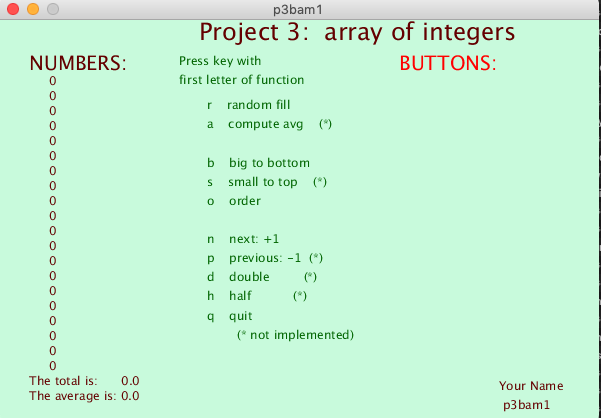## PROJECT 3:   Array of integersClick to enlarge.
 Create an integer array named "z"     containing at least twenty (20) integer values. Display the contents of the array, Also display sum total & mean average When a button or key is pressed     modify the array (as described below).

• Write a (void) function to display the contents of the array, one element per line.

• Compute and display the sum total and the mean average of all elements in the array.

• Write functions to modify or manipulate ANY array of integers, performing operations as described in the table below.

• Add buttons as indicated below; add code to mousePressed() to call each function when the corresponding button is clicked.
 ```int many=20; int[] z = new int[many]; float total, average; ```

Your draw() function should look something like this:

 ```void draw( ) { background( 200,255,200 ); scene(); // Show the buttons. display( z, many ); // Show the array. messages(); // Title, author, average } ```

Everything else is done by "closed" functions that are called when a key or button is pressed.

Each function should accept two arguments:

• an array of integers
• the size of the array.
NOTE:   Do not use global variables to refer to the array and size, in these "closed" functions.   Instead, use the arguments, as in the following examples:

Here is an example of a function that displays an array:
 ```// Display a list of array values void display( int[] v, int m ) { int x=50, y=60, spacing=15; textSize( 12 ); for (int i=0; i

This function returns the sum of the elements:
 ```// Compute the sum. float sum( int[] w, int m ) { float result=0; for (int j=0; j

These functions are called

• when a key is pressed, OR . . .
• when a button is clicked.
Action Key Button
void reset( int a[], int m )
// Fill the array with random values
// from zero to 999.
r RESET
void calc( int a[], int m )
// Calculate new total and average.
c CALC
void next( int a[], int m )
// Increase each of the numbers by one.
n NEXT
void dbl( int a[], int m )
// Double each of the numbers.
d DOUBLE
void half( int a[], int m )
// Reduce each by half.
h HALF
void big( int a[], int m )
// Move biggest number to end of the array.
// (Swap elements, to preserve all numbers.)
b BIG
void order( int a[], int m )
// For extra credit, rearrange (sort) the entire array (in ascending order).
NOTE: Do not use the name "sort for this function. (That is a reserved word, in Processing!)
o ORDER
void var( int a[], int m )
// For extra credit, compute the variance and standard-deviation.
v SIGMA

NOTE:   Do not use global values in these functions; use the arguments.

KEYS   The keyPressed() function should have code to call a function when the key is the first letter of that function.
 ``` r reset() // Replace with random values. c calc() // Calculate new sum and average. n next() // Increase each by one. d dbl() // Double each of the numbers. h half() // Reduce each by half. b big() // Move biggest to end of array. o order() // Rearrange array in ascending order. (sort) ```

BUTTONS
create buttons with names for each of these,
and add mousePressed() code to call these functions when button is pressed.